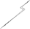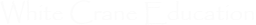Classrooms Curricula References Textbooks Site Map
 Our classrooms section has a variety of free classrooms for use by students and their teachers. Our Short Courses are quick reviews of material that you'll find in other classes such as using linear equations and working with polynomials. They contain multiple examples and lists of steps for common procedures and have our signature dynamic practice areas that generate an almost unlimited number of practice questions along with their complete solutions. The classes in the Supplemental Curriculum take students through material not normally convered in a middle school or high school curriculum through a series of lectures that include real world applications and interactive modules.Short Course Supplemental Curriculum

# General MathematicsThis short course covers general topics from introductory mathematics and arithmetic such as fractions and percentages.

# Linear EquationsLinear equations are an important part of every algebra class. Not only do they illustrate many basic algebra principles but they're a basic tool in mathematical modeling. They can be used to describe everything from the motion of cars to the cost of manufacturing processes.

# PolynomialsPolynomials are one of the building blocks of engineering. They can be used to model everything from the behavior of falling objects to complex dynamic systems. Our short course covers classification, addition and subtraction, multiplication, factoring and solving equations including the Quadratic Equation.

# Rational ExpressionsRational expressions are just fractions where the numerator and denominator are polynomials, i.e. they have variables rather than just numbers. That may sound simple enough but, when you start trying to work with them, they can turn out to be quite tricky. Not because the concepts are difficult but because there are a lot of calculations involving multiple polynomials that can quickly become very messy. This short course takes you, step by step, through all of the basic procedures you need to know to work with these objects.